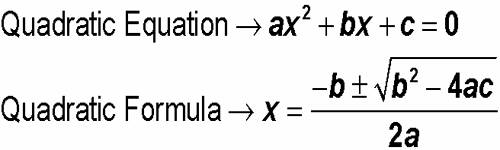A polynomial of the second degree is generally called a quadratic polynomial. In elementary algebra, the quadratic formula is the solution of the quadratic equation. There are other ways to solve the quadratic equation instead of using the quadratic formula, such as factoring, completing the square, or graphing. Using the quadratic formula is often the most convenient way.

If f(x) is a quadratic polynomial, then f(x) = 0 is called a quadratic equation.

An equation in one unknown quantity in the form ax2 + bx + c = 0 is called quadratic equation.The theory of quadratic equation formulae will help us to solve different types of problems on the quadratic equation.

The general form of a quadratic equation is ax2 + bx + c = 0 where a, b, c are real numbers (constants) and a ≠ 0, while b and c may be zero.

(i) The Discriminant of a quadratic equation is ax2 + bx + c = 0 (a ≠ 0) is ∆ = b2 – 4a

(ii) If α and β be the roots of the equation ax2 + bx + c = 0 (a ≠ 0) then

α + β =  – b/a = – [coefficient of x / coefficient of x2]

and αβ = c/a = [constant term / coefficient of x2]

(iii) The formula for the formation of the quadratic equation whose roots are given: x2 – (sum of the roots)x + product of the roots = 0.

(iv) When a, b and c are real numbers, a ≠ 0 and discriminant is positive (i.e., b2 – 4ac > 0), then the roots α and β of the quadratic equation ax2 + bx + c = 0 are real and unequal.

(v) When a, b and c are real numbers, a ≠ 0 and discriminant is zero (i.e., b2 – 4ac = 0), then the roots α and β of the quadratic equation ax2 + bx + c = 0 are real and equal.

(vi) When a, b and c are real numbers, a ≠ 0 and discriminant is negative (i.e., b2 – 4ac < 0), then the roots α and β of the quadratic equation ax2 + bx + c = 0 are unequal and imaginary. Here the roots α and β are a pair of the complex conjugates.

(viii) When a, b and c are real numbers, a ≠ 0 and discriminant is positive and perfect square, then the roots α and β of the quadratic equation ax2 + bx + c = 0 are real, rational unequal.

(ix) When a, b and c are real numbers, a ≠ 0 and discriminant is positive but not a perfect square then the roots of the quadratic equation ax2 + bx + c = 0 are real, irrational and unequal.

(x) When a, b and c are real numbers, a ≠ 0 and the discriminant is a perfect square but any one of a or b is irrational then the roots of the quadratic equation ax2 + bx + c = 0 are irrational.

(xi) Let the two quadratic equations are a1x2 + b1x + c1 = 0 and a1x2 + b2x + c2 = 0

Condition for one common root: (c1a2 – c2a1)2 = (b1c2 – b2c1)(a1b2 – a2b1), which is the required condition for one root to be common of two quadratic equations.

Condition for both roots common: a1/a2 = b1/b2 = c1/c2

(xii) In a quadratic equation with real coefficients has a complex root α + iβ then it has also the conjugate complex root α – iβ.

(xiii) In a quadratic equation with rational coefficients has an irrational or surd root α + √β, where α and β are rational and β is not a perfect square, then it has also a conjugate root α – √β.

Information Source;